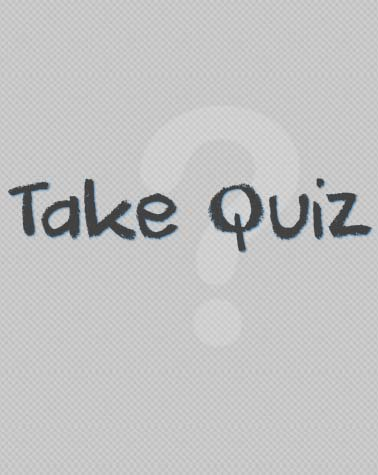# Quiz - 2 Codegeeks

16 Questions | Total Attempts: 75SettingsCreate your own Quiz• 1.
What is output: printf("%d" printf("hello") );
• A.

Hello

• B.

Hello0

• C.

Hello5

• D.

None

• 2.
What is output: printf( "%d", printf('' %d" , printf("hii"));
• A.

Hi31

• B.

Hii31

• C.

Hii

• D.

None

• 3.
What is output: int a = 10, b = 20, c = 30; printf("%d %d %d");
• A.

102030

• B.

30 20 10

• C.

10

• D.

Any value between 10 , 20 , 30

• 4.
What is the output: printf(" %d " , printf("%dhii", printf("hello")));
• A.

Hellohi53

• B.

Hello5hii4

• C.

Hellohi

• D.

Hello5hii1

• 5.
In which line there is an error in the program : 1 int main() 2 { 3    char ch; 4   scanf("%s", ch); 5    printf("%s", ch); 6    return 0; 7 }
• A.

1

• B.

2

• C.

4

• D.

5

• 6.
What is the value of num? int num = 4/3*2.4;
• A.

3

• B.

3.33

• C.

2

• D.

2.0

• 7.
What is the value of the num: int num = -17 % 4;
• A.

1

• B.

4

• C.

-1

• D.

-4

• 8.
What is the value of the num: int num = 17 % -4;
• A.

-1

• B.

1

• C.

4

• D.

-4

• 9.
What is the output of the following program? int num = 10; while ( num < 12 ) {     cout << num;     num++; }
• A.

101112

• B.

1011

• C.

1112

• D.

None

• 10.
What is the output : int num = 10; while(num < 12 ); {      cout << num ;      num++; }
• A.

Error

• B.

Nothing is printed on console

• C.

1011

• D.

2111

• 11.
What is the output: int num = 10; while( num < 10 ) {      cout << num;      num++; }
• A.

Error

• B.

1011

• C.

109

• D.

Nothing is printed on the screen

• 12.
What is output  #include<stdio.h> int main() {       int a = 500, b, c;       if(a>=400)            b = 300;       c = 200;       printf("%d %d", b, c); }
• A.

Grbage_value 200

• B.

300 200

• C.

200 300

• D.

300 grbage_value

• 13.
What is output of following program: #include< stdio.h> int main() {      int i = 65;      char j = 'A';      if( i == j )            printf("C is WOW \n");      else              printf(" C is headache \n");        return 0;    }
• A.

• B.

C is WWO

• C.

C is WOW

• D.

Nothing

• 14.
IN which line there is an error  1 #include <stdio.h> 2 int main() 3 { 4     int x = 10, y = 15;      (x + 1) = 15; 5     if( x%2 == y%3) 6             printf("Carpathians\n"); 7     8 }
• A.

4

• B.

5

• C.

2

• D.

7

• 15.
What will be the output of the following program? #include int main() {        int i = 4, j = -1, k = 0, w,  x, y, z;        w = i || j || k;        x = i && j && k;        y = i || j && k;         z = i && j || k;        printf(" w = %d x = %d y = %d z = %d \n" , w, x, y,z);       return 0; }
• A.

W = 0 x = 1 y == 1 z =1

• B.

W = 1 x = 0 y == 1 z =1

• C.

W = 0 x = 1 y == 1 z =0

• D.

W = 0 x = 1 y == 0 z =1

• 16.
What is the output: #include< stdio.h > int main() {      int n =9;      ( (n == 9.5) ? printf(" Correct \n ") : printf(" wrong \n") );       return 0; }
• A.

Correct

• B.

Wrong

• C.

Correct wrong

• D.

None

Related TopicsBack to top
×

Wait!
Here's an interesting quiz for you.